# Tag: Technical Indicator

## What is the Arnaud Legoux Moving Average and how to use it on Tuned?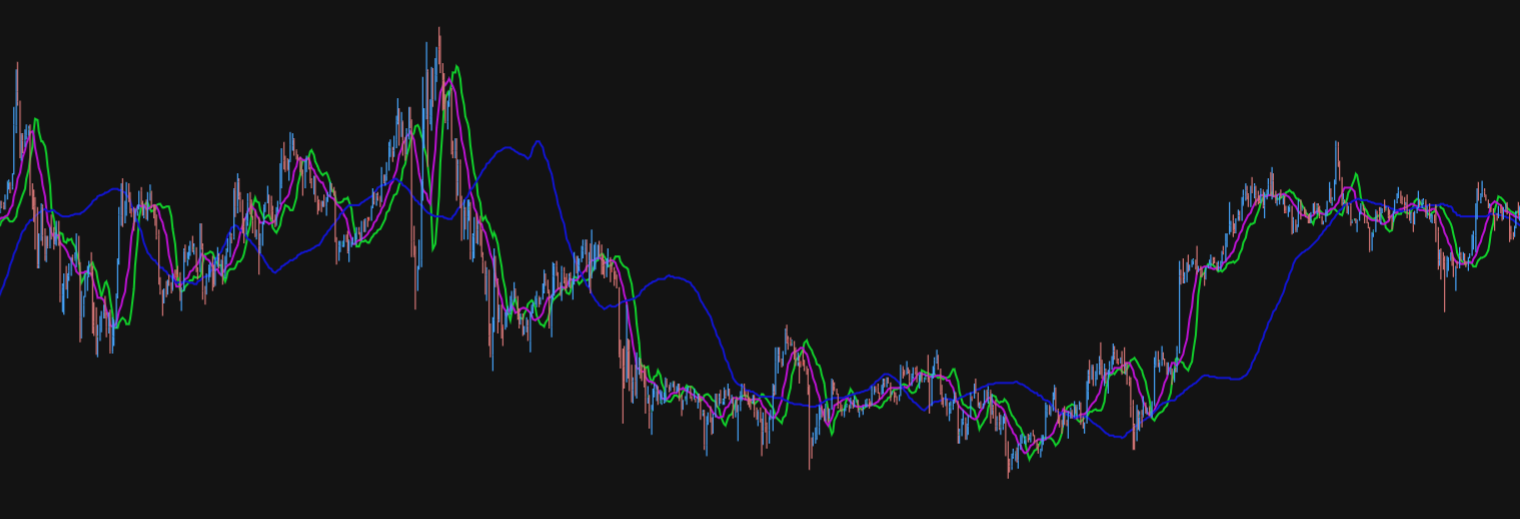In this article, we’ll be exploring what the Arnaud Legoux Moving Average (ALMA) indicator is, what it measures, common uses, and how it can be applied to Tuned and algorithmic trading in general.

## What is the Williams %R indicator and how to use it on Tuned?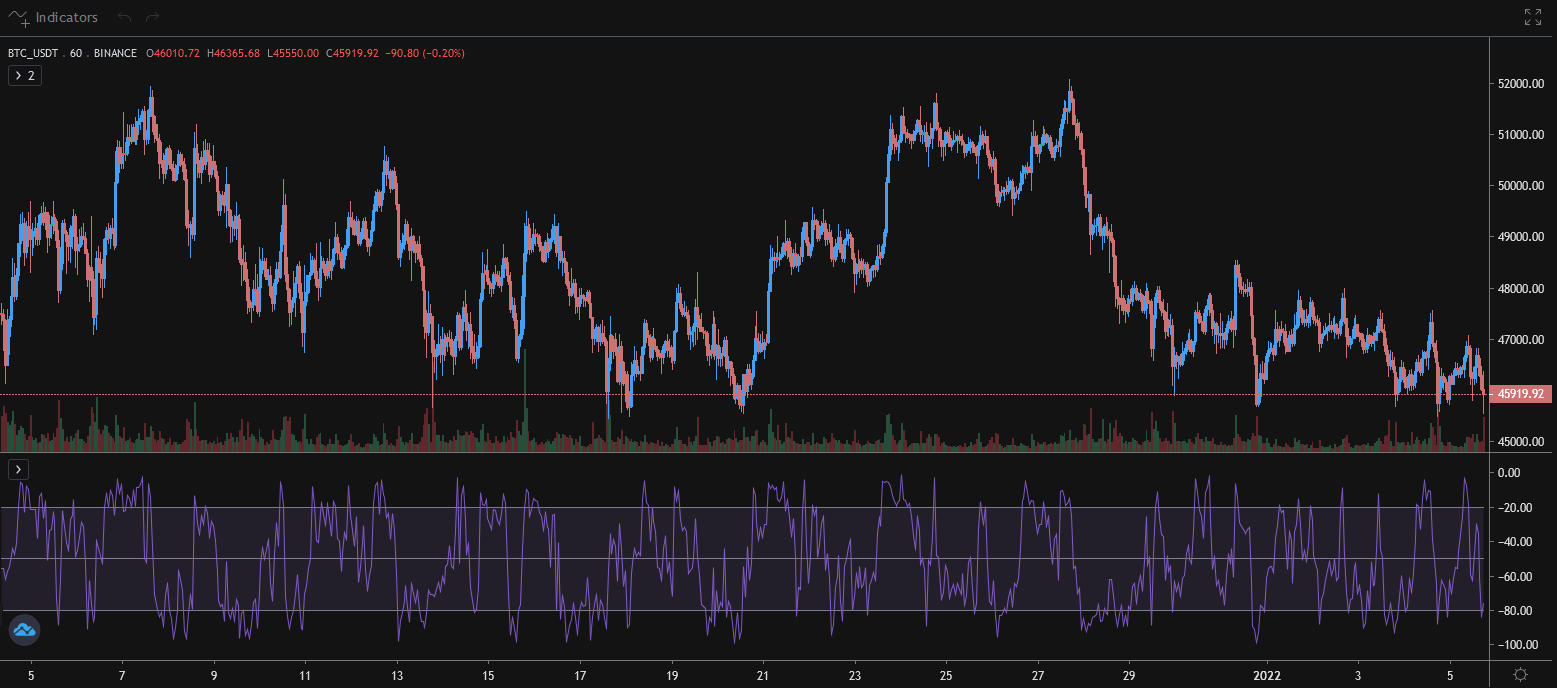We will look at what it does, how it’s used, and how it can be utilized on Tuned for algorithmic trading.

## What is the On-Balance Volume indicator and how to use it on Tuned?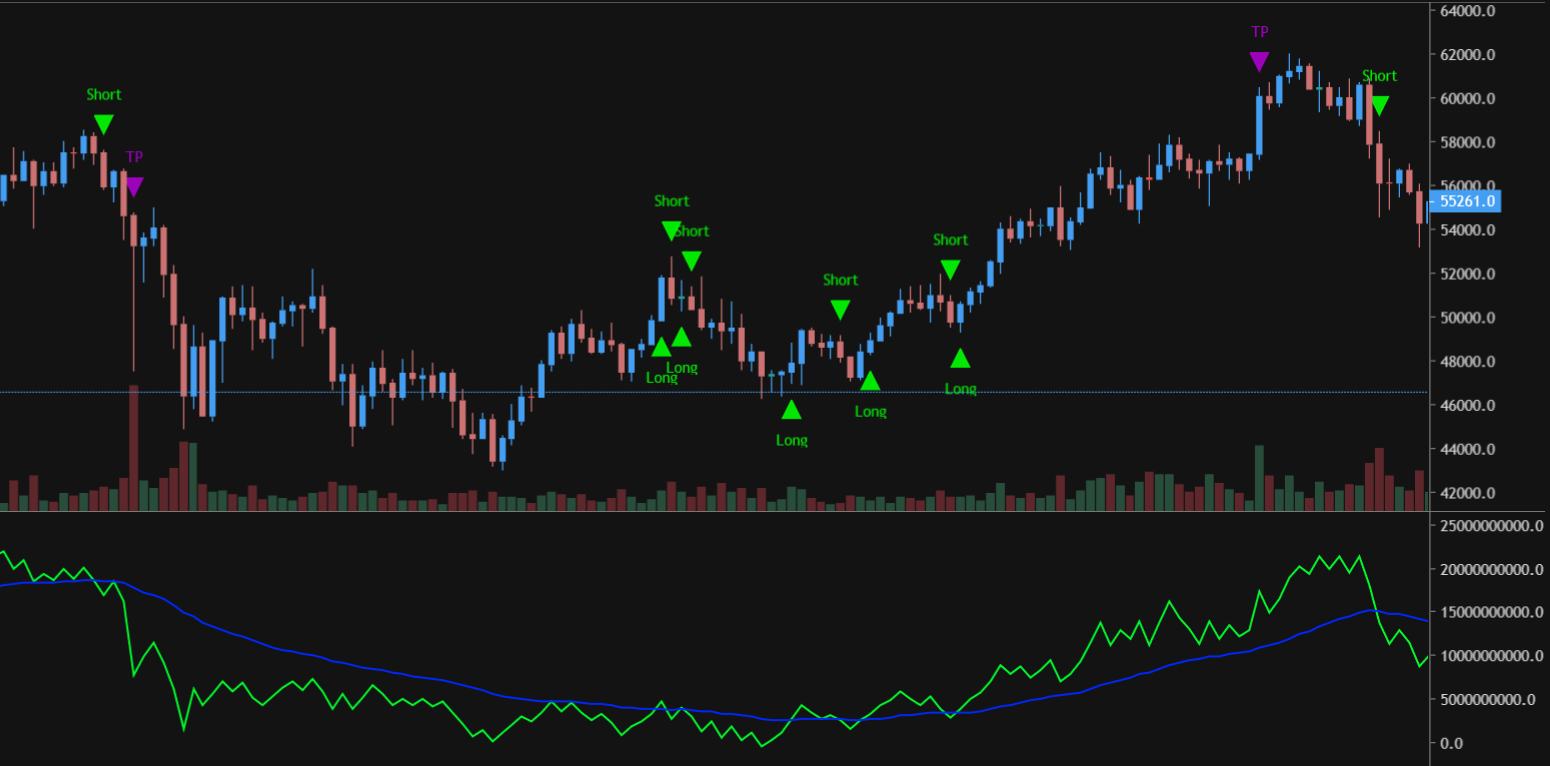In this article, we’ll be exploring what the On-Balance Volume (OBV) indicator is, what it measures, common uses, and how it can be applied to Tuned and algorithmic trading in general.

## What is the Momentum Indicator and how to use it on Tuned?In this article, we’ll be talking about the Momentum indicator.We’ll be exploring what it does, its common uses and how it can be applied to Tuned and algorithmic trading in general. Table of contents What is the Momentum Indicator? How is Momentum calculated? Common uses An example strategy using Momentum on Tuned Conclusion What is…

## What is the Rate of Change indicator and how to use it on Tuned?In this article, we’ll be exploring what the Rate of Change (RoC) is, what it measures, common uses and how it can be applied to Tuned and algorithmic trading in general.

## What is CCI and How to Use It in Tuned Script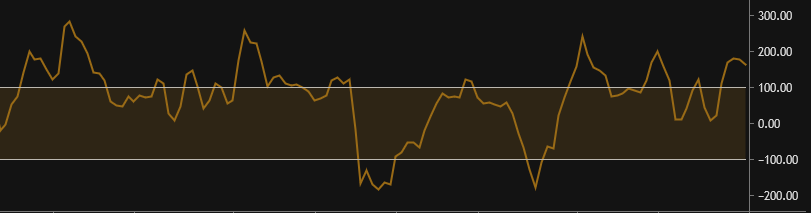What is the Commodity Channel Index (CCI)? Key points about the CCI How to add the CCI to my script? How to use the CCI to generate buy and sell signals? What Is the Commodity Channel Index(CCI)? The CCI is a momentum-based oscillator used to help determine when an asset is reaching a condition of…

## What is MACD and How to Use It in Tuned ScriptWhat Is Moving Average Convergence Divergence (MACD)? Moving Average Convergence Divergence (MACD) is a trend-following momentum indicator that shows the relationship between two moving averages of an assets price. The MACD is calculated by subtracting the 26-period exponential moving average (EMA) from the 12-period EMA. The outcome of that calculation is the MACD line. A 9-period EMA of…

## What is RSI and How to Use It in Tuned Script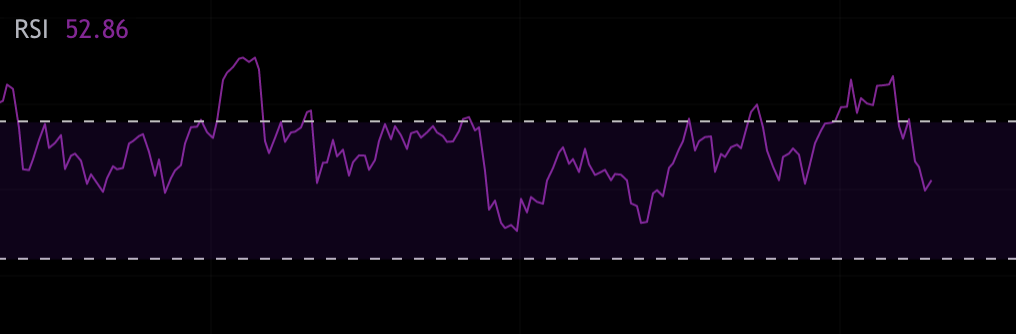What Is the Relative Strength Index (RSI)? The relative strength index (RSI) is a momentum indicator used in technical analysis that measures the magnitude of recent price changes to evaluate overbought or oversold conditions in the price of a stock or other asset. The RSI is displayed as an oscillator (a line graph that moves…

## What are Moving Averages and How to Use Them in Tuned Script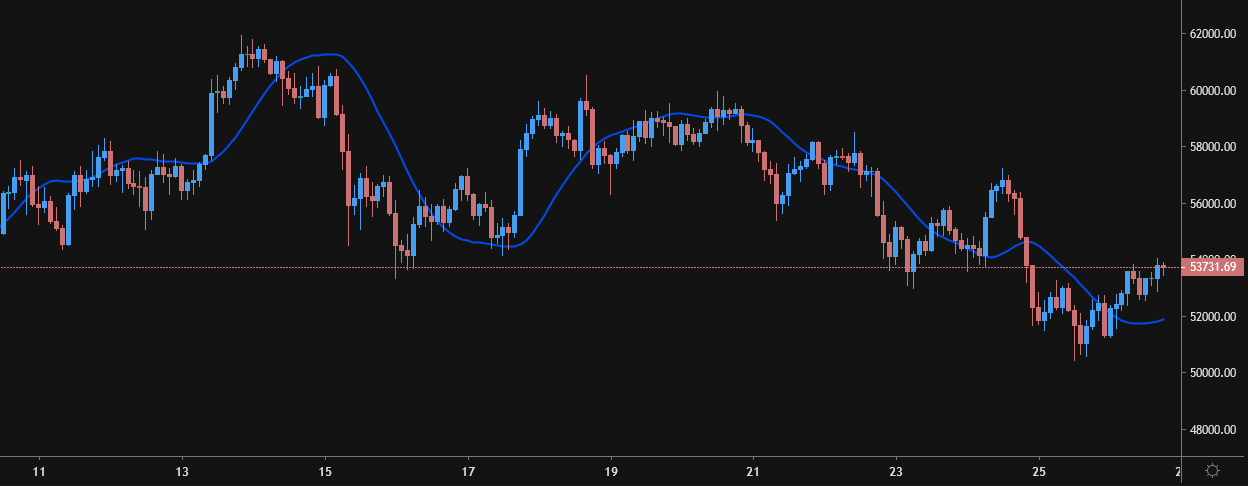What Is a Moving Average (MA)? In statistics, a moving average is a calculation used to analyze data points by creating a series of averages of different subsets of the full data set. In finance/crypto, a moving average (MA) is an indicator that is commonly used in technical analysis. The reason for calculating the moving…

### Tags:

All investing involves risk, including the possible loss of all the money you invest, and past performance does not guarantee future performance. Historical returns expected returns, and probability projections are provided for informational and illustrative purposes, and may not reflect actual future performance.

Tuned is not a broker-dealer, exchange, custodian, wallet provider, or counterparty. Investing is highly speculative and volatile. Tuned is only suitable for investors who fully understand the risk of loss and may experience large drawdowns. Investors should never invest more than they can afford to lose.